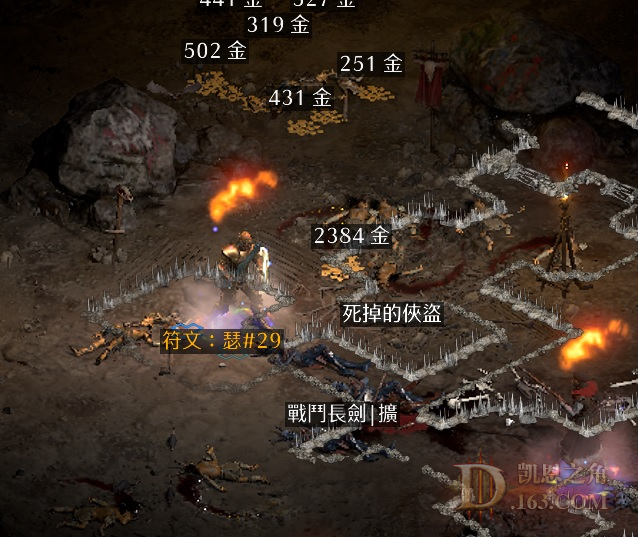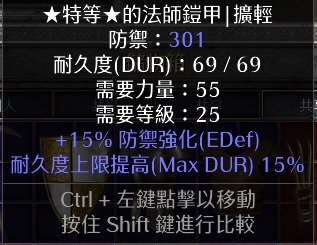//没登陆

 下午第一把地穴出货了，超市啥都不出wlwsor#3155

24=2 25=7 26=2 27=2 28=1 29=3 30=1
31=2 32=1 33=1

24=2
Item：15 15 mp+  200/9 wxtt
 可以的，法凯26+25-27

 赞啊。我两天连出31 + 29 *2 ，发现运气好了要连续作战。

dancer#11185
30# 法师 KP
31# 亚马逊 地穴
29# 亚马逊 KC *2
33# 亚马逊 chaos
 6666666666666666666666666666666666

 发誓铠甲防御这么低啊

 今天出货22#  28#wlwsor#3155

24=2 25=7 26=2 27=2 28=1 29=3 30=1
31=2 32=1 33=1

24=2
Item：15 15 mp+  200/9 wxtt
 不想肝了 发表于 2022-5-13 15:23 今天出货22#  28#你看，我这个有个19/18的骑士火炬。。。要不要考虑下